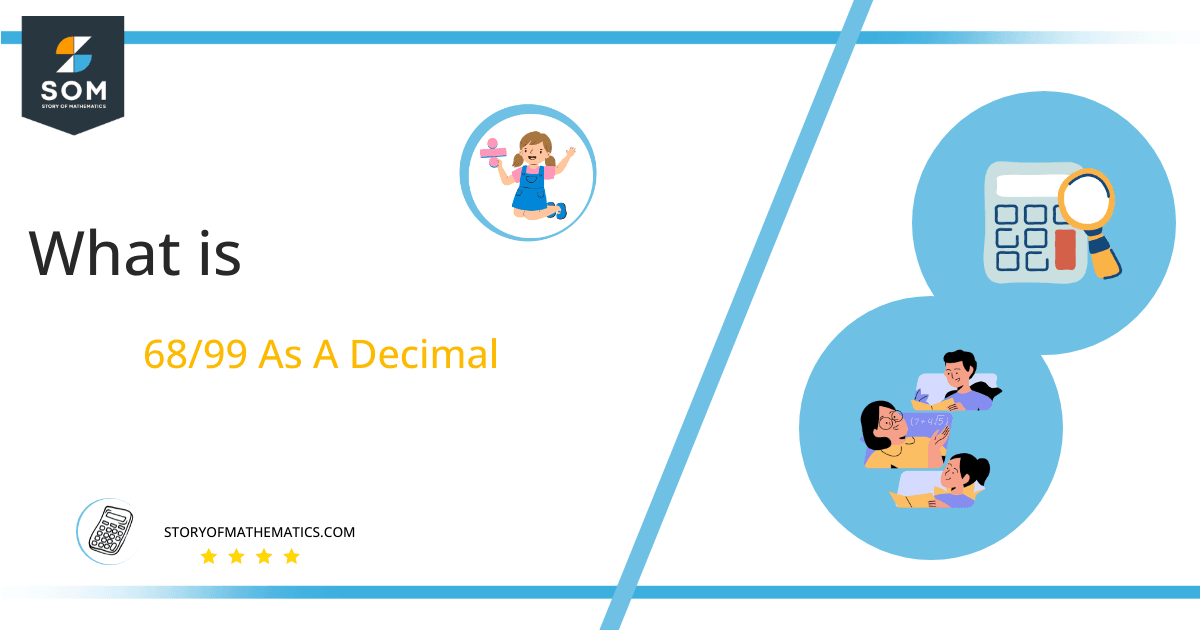#What Is 68/99 as a Decimal + Solution With Free Steps

The fraction 68/99 as a decimal is equal to 0.686.

Fractions act as an alternate, compact notation for the division of two numbers and q, where p and q are respectively called the numerator and denominator. Unlike the usual notation p $\boldsymbol\div$ q, a fraction looks like p/q, where the “/” replaces the “$\div$” symbol.Here, we are more interested in the division types that result in a Decimal value, as this can be expressed as a Fraction. We see fractions as a way of showing two numbers having the operation of Division between them that result in a value that lies between two Integers.

Now, we introduce the method used to solve said fraction to decimal conversion, called Long Division, which we will discuss in detail moving forward. So, let’s go through the Solution of fraction 68/99.

## Solution

First, we convert the fraction components, i.e., the numerator and the denominator, and transform them into the division constituents, i.e., the Dividend and the Divisor, respectively.

This can be done as follows:

Dividend = 68

Divisor = 99

Now, we introduce the most important quantity in our division process: the Quotient. The value represents the Solution to our division and can be expressed as having the following relationship with the Division constituents:

Quotient = Dividend $\div$ Divisor = 68 $\div$ 99

This is when we go through the Long Division solution to our problem.Figure 1

## 68/99 Long Division Method

We start solving a problem using the Long Division Method by first taking apart the division’s components and comparing them. As we have 68 and 99, we can see how 68 is Smaller than 99, and to solve this division, we require that 68 be Bigger than 99.

This is done by multiplying the dividend by 10 and checking whether it is bigger than the divisor or not. If so, we calculate the Multiple of the divisor closest to the dividend and subtract it from the Dividend. This produces the Remainder, which we then use as the dividend later.

Now, we begin solving for our dividend 68, which after getting multiplied by 10 becomes 680.

We take this 680 and divide it by 99; this can be done as follows:

680 $\div$ 99 $\approx$ 6

Where:

99 x 6 = 594

This will lead to the generation of a Remainder equal to 680 – 594 = 86. Now this means we have to repeat the process by Converting the 86 into 860 and solving for that:

860 $\div$ 99 $\approx$ 8

Where:

99 x 8 = 792

This, therefore, produces another Remainder which is equal to 860 – 792 = 68. Now we must solve this problem to Third Decimal Place for accuracy, so we repeat the process with dividend 680.

680 $\div$ 99 $\approx$ 6

Where:

99 x 6 = 594

Finally, we have a Quotient generated after combining the three pieces of it as 0.686, with a Remainder equal to 86.Images/mathematical drawings are created with GeoGebra.